# Apple Numbers Formulas: How To Use Them Effectively

Jun 11, 2022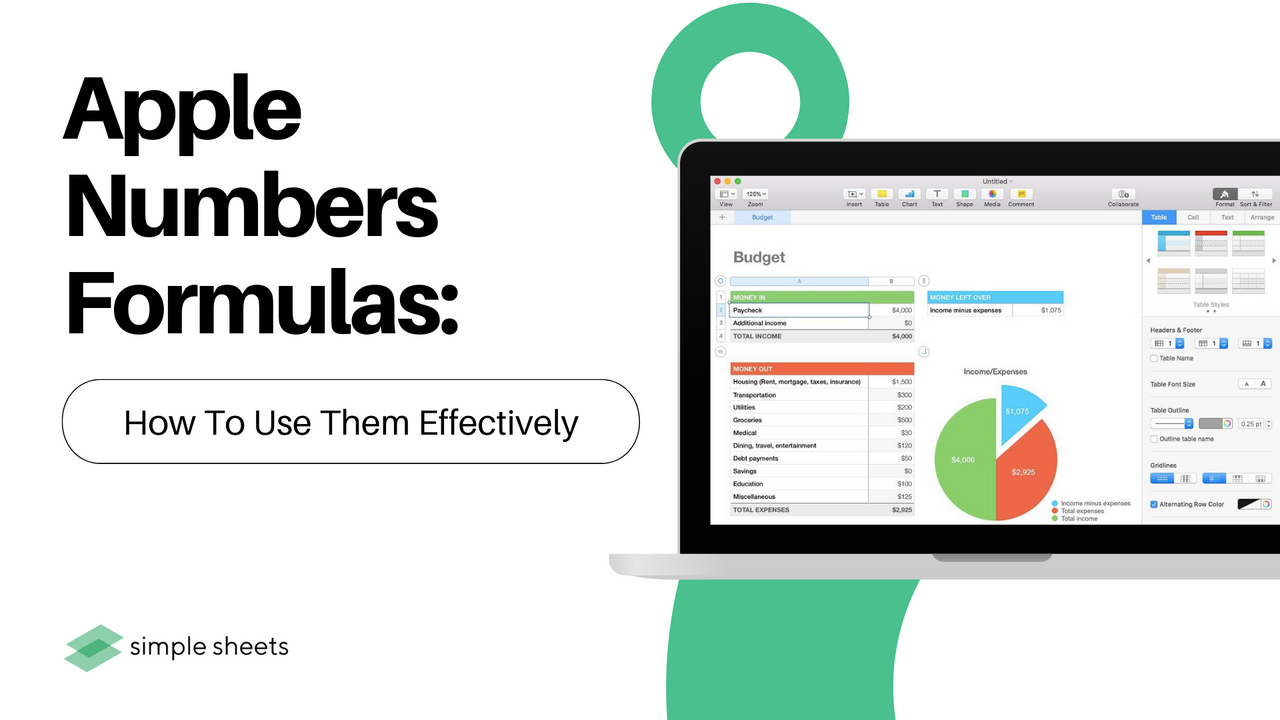Do you use Apple Numbers on your macOS? Discover how you can use formulas and functions to make your data much easier to work with.

Put the old calculator away and stop taking ages, inputting data, and analyzing it manually. This article will show you how to use formulas and functions within Numbers quickly and easily using built-in tools.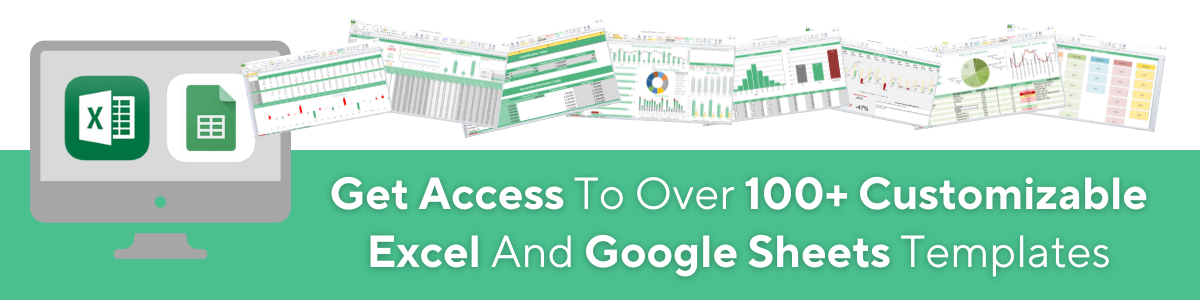## Quick and Easy Formulas

Apple Numbers provides some basic formulas to test out quickly for those who do not class themselves as spreadsheet experts.

Choose a cell reference where you want to place your formula. Hit the Insert button from the toolbar and select the sum, minimum, average, count, maximum, or product.

Based on the data you have input into the spreadsheet, Numbers will guess which cells you want to use and highlight the cell range for you. If you are unhappy with Numbers' choices, you can amend them.

Select the cell containing your formula, and the Formula editor will appear. Choose the correct cells and select the checkmark.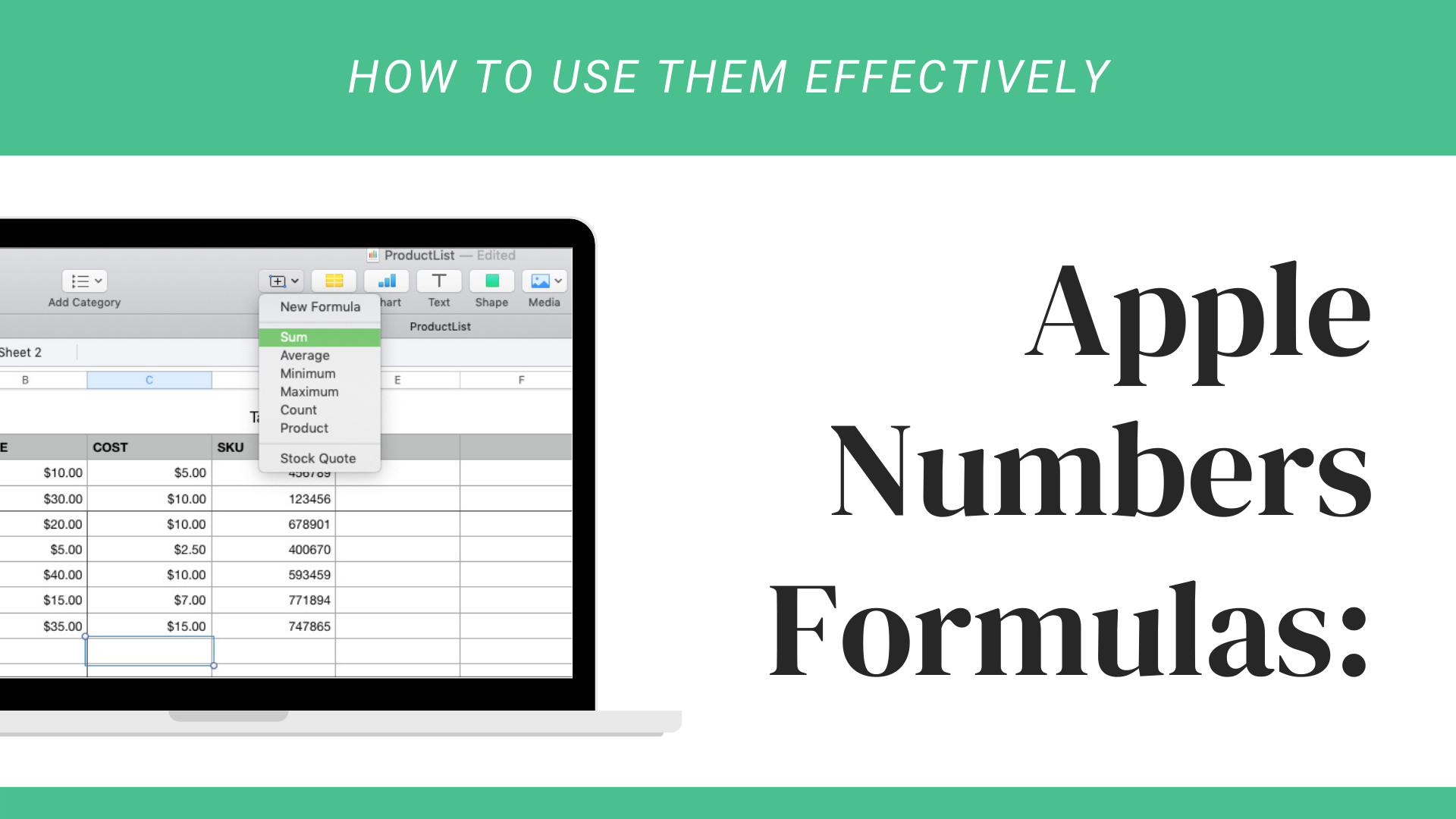## More Useful Formulas and Functions

### Compare Values.

You can use a comparison operator to compare values and check if they are more significant than, equal, less than, or not equal.

The results within the formula will show as True or False. This tool compares sales, expenses, or income over any given period.

### Split Text.

You can break up text strings if you require more information or need to gather specific data via the Right and Left functions.

This function is fantastic for gathering anything from phone numbers, states, or zip codes from address strings.## Easier Ways to Analyze Data in Numbers

Using formulas and functions in Numbers is a much easier way to manipulate or analyze the data. To deal with numbers, try out different formulas in numbers. You might find a new formula that perfectly suits your needs!

### Can you use formulas in Apple numbers?

You can access all formulas in numbers via the equal sign. Choose any mathematical function you need and where you want the corresponding data to appear in your sheet, just as you would in Microsoft excel. Numbers spreadsheets have all the functions you would expect to see.

### Where are the formulas in Apple numbers?

Head into the Apple Numbers App.

Select the + icon from the top right-hand corner of the screen.

Select the Blank Template option.

From here, submit the values in their cells and choose the cell where your calculated result appears.

Press the equal sign from the keyboard to show all formulas in numbers.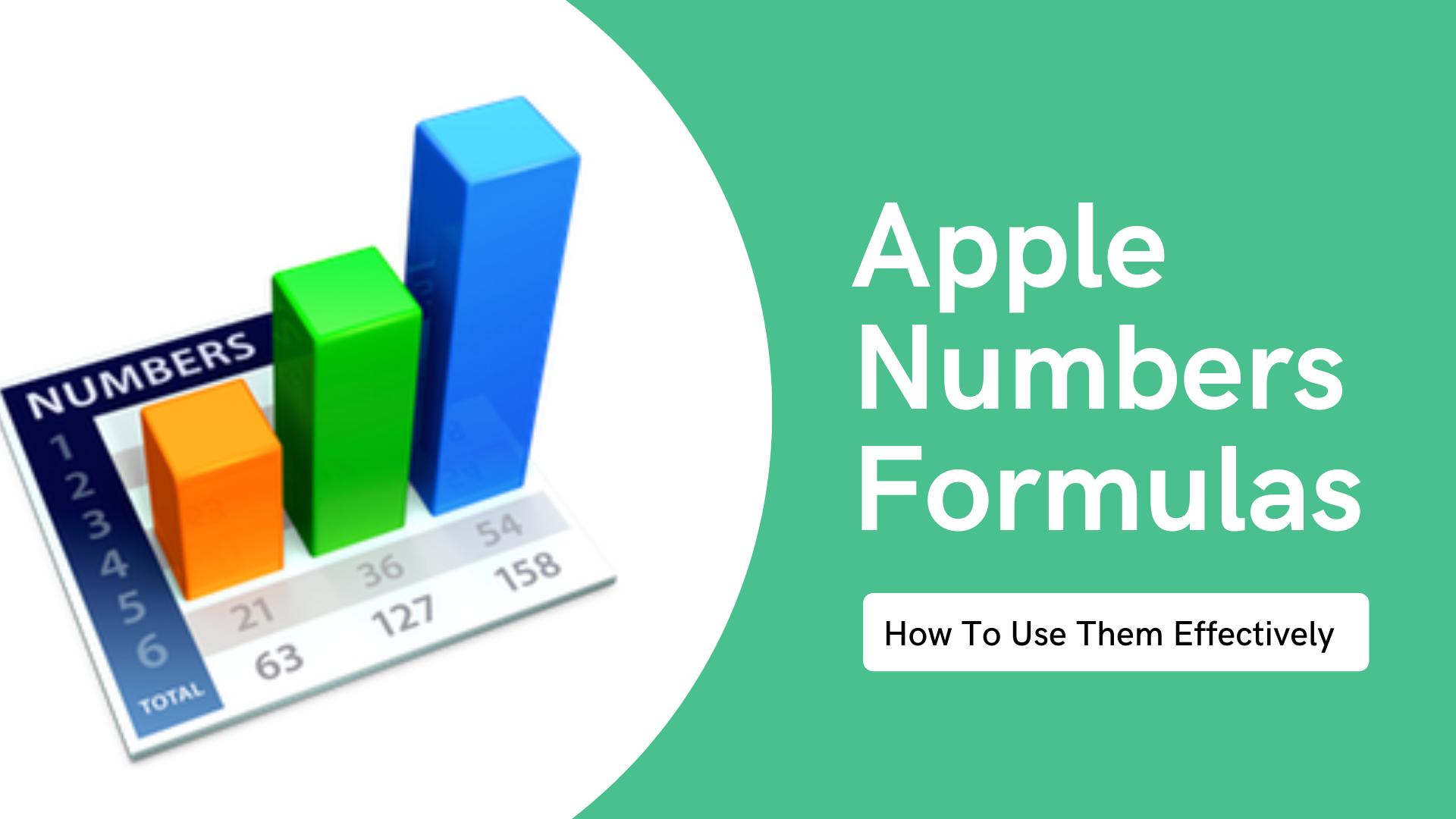### Do Apple numbers have a formula bar?

You can access formulas straight from your iPad, Apple Mac, or iPhone using Apple Numbers spreadsheets. These functions allow you to compare values in multiple rows, perform basic arithmetic, and create formulas quickly and easily.

### How do I apply a formula to an entire column in numbers?

If you would instead not use a keyboard shortcut to create a new formula in Numbers spreadsheets, you formulate the Fill feature to apply any formula in Numbers to an entire column or row.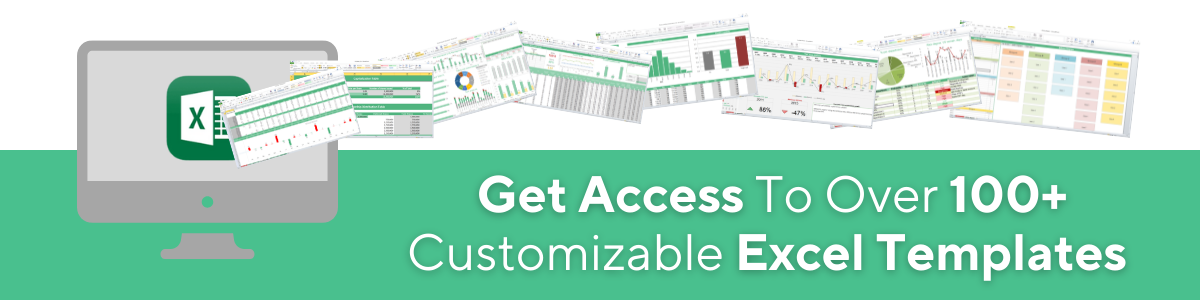## Related Articles:

What is Apple Numbers For Mac: Everything You Need to Know

Microsoft Excel is Waiting for Another Application to complete an OLE Action - What Does This Mean?